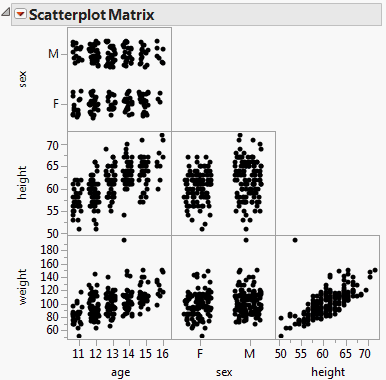Essential Graphing > Scatterplot Matrix > The Scatterplot Matrix Window
Publication date: 04/12/2021

# The Scatterplot Matrix Window

The Scatterplot Matrix window shows an ordered grouping of bivariate graphs. In each graph, you can examine the relationships between each pair of variables.

Follow the instructions in Example of a Scatterplot Matrix to produce the plot shown in Figure 6.6.

Note: For information about additional options, see Scatterplot Matrix Platform Options.

Figure 6.6 Example of a Scatterplot Matrix WindowReplace variables in the plot by dragging and dropping a variable, in one of two ways: swap existing variables by dragging and dropping a variable from one axis to the other axis; or, click a variable in the Columns panel of the associated data table and drag it onto an axis. This feature is not available for matrices in the Square format.

Want more information? Have questions? Get answers in the JMP User Community (community.jmp.com).